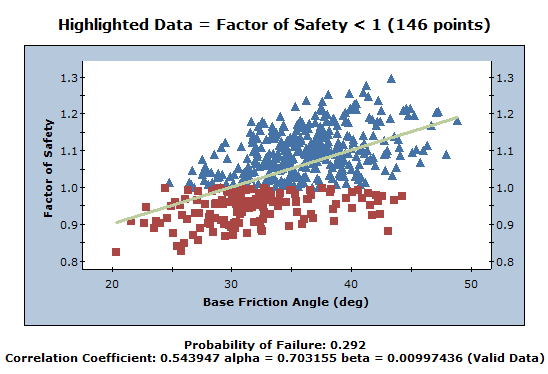# Scatter Plot

To create a scatter plot after a Probabilistic analysis:

1. Select Scatter Ploton the toolbar or the Statistics menu to open the Scatter Plot dialog.2. Select the data you want to plot on the X-axis (X Axis Dataset) and y-axis (Y Axis Dataset).
3. To highlight data in the plot with a Factor of Safety lower than a certain number, select the Highlight Data check box and enter a value ( the default = 1).
4. Click OK.
The Scatter Plot is generated in the Scatter Plot View.## Regression Line

If the Regression Line option is selected in the right-click menu, you will see a listing of Correlation Coefficient, alpha and beta listed at the bottom of the Scatter Plot as described below.

## Correlation Coefficient

The Correlation Coefficient indicates the degree of correlation between the two variables plotted. The Correlation Coefficient can vary between -1 and 1 where numbers close to zero indicate a poor correlation, and numbers close to 1 or -1 indicate a good correlation. Note that a negative correlation coefficient simply means that the slope of the best fit linear regression line is negative.

## Alpha and Beta

Alpha and beta represent the y-intercept and slope, respectively, of the best fit linear regression line to the scatter plot data. The linear regression line can be displayed on the plot with the Regression Line option in the right-click menu.

## Right Click Options

The following options are available in the Scatter Plot View right-click menu:

• Regression Line
• Sampler
• Chart Properties
• Change Plot Data
• Copy
• Copy Data
• Plot in Excel
• Export Image